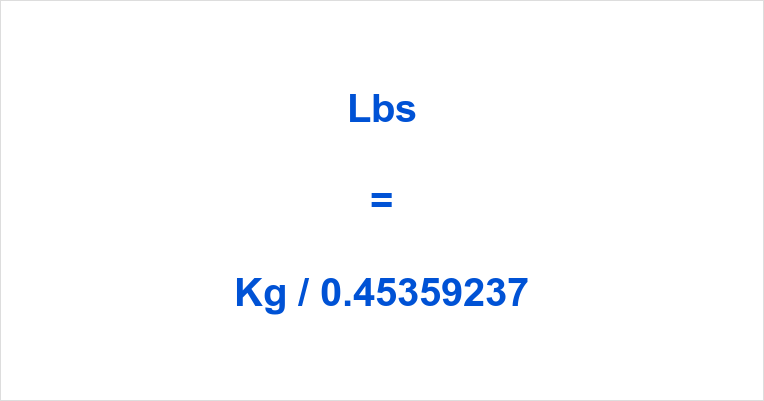# LBS to KG

## LBS to KG

### 1 LB = 0.453592 KG

The kilogram is the base unit of mass in the (SI) International System of Units, commonly called the metric system.

In turn, the pound is an imperial unit and United States customary unit.

This pound-mass is a also referred to as avoirdupois pound.

The unit symbol for kilogram is kg, whereas the mass pound is usually abbreviated as lb (derived from the Roman libra), lbm or lbm.

Since 1959, the international avoirdupois pound is legally defined as 0.45359237 kg, that is 16 ounces.

Therefore, to convert kg to lbs you have to divide the mass in pounds by 0.45359237.

The kg to lbs formula is: [lb] = [kg] / 0.45359237

For example, to convert 2 kilograms to pounds divide 2 by 0.45359237 to obtain ~ 4.41 lbs.

If you want convert kilograms to pounds easier, then use our kg to pounds converter above.

Our tool converts the units of mass, kilos to lbs, as you type.

## Converter Kg to Lbs

Our kilos to pounds converter at the top of this page uses a decimal point for fractions. Changing the lower field swaps the units.

Instead of using our kg to pound converter you may also search for frequent kilo to pound conversions by means of the form in the sidebar.

Check it out now:

With x being your quantity type, for instance, x kilogram to pounds, or write x kg to lbs.

Alternatively, you can enter x kilos into pounds, or x kilogram to pound.

## How many Pounds in a Kilogram

Different definitions of (mass) pound have been used in the course of history, but these days one avoirdupois pound is defined as 0.45359237 kilograms.

Thus, the answer to the questions how many lb in a kg and how many pounds in a kilogram is exactly 1 / 0.45359237 kg.

1 kg ~ 2.20 lb ⇔ 1 kg ~ 2.20 lbm ⇔ 1 kg ~ 2.20 lbm

Spelled out, one kilogram is approximately equal to two point two pounds.

As an estimate, you can think of a kilogram as being equal to 2.2 international avoirdupois pounds.Frequent conversions include, for example:

If you like to know how to change kg to pounds for Troy, Tower, London, Merchant, Byzantine and Metric pounds then check out the formulas below and/or see the referenced Wiki article.

• London pounds = [kg] / 0.466552152
• Merchants’ pounds = [kg] / 0.4373926425
• Metric pounds = [kg] * 2
• Tower pounds = [kg] / 0.349914114
• Troy pounds = [kg] / 0.3732417216

MG to mL